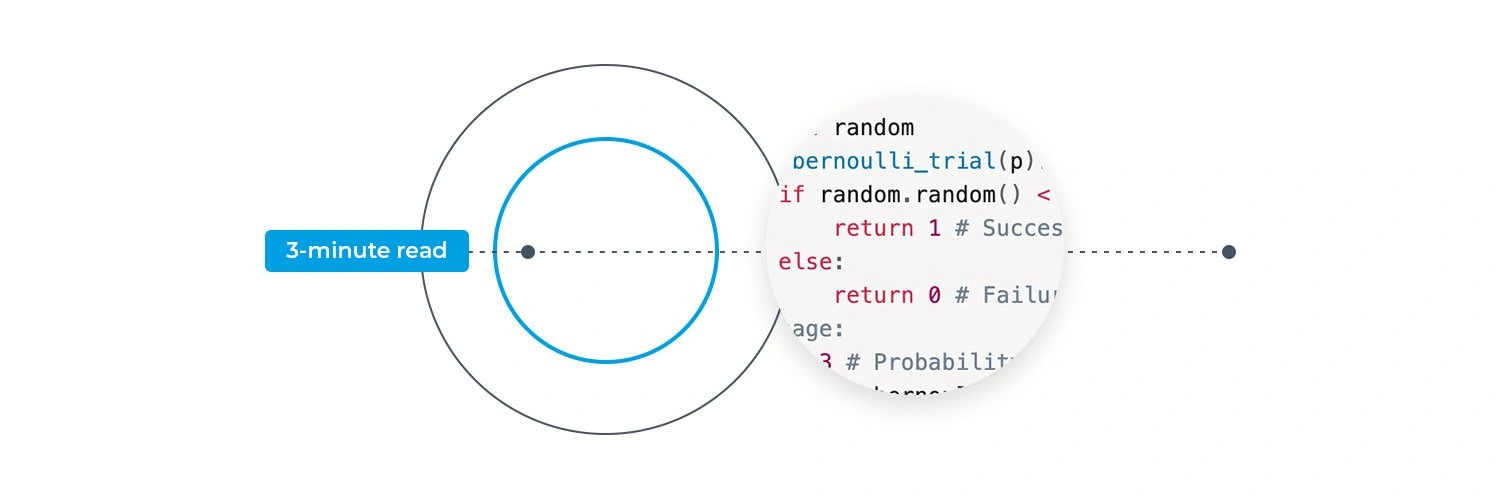# Bernoulli Distribution & Its Uses

### Author: Daragh Ó Tuama

Bernoulli Distribution & Its UsesThe Bernoulli distribution plays a pivotal role in probability theory and statistics. Named after the Swiss mathematician Jacob Bernoulli, this distribution forms the foundation of many statistical models and serves as a fundamental concept in various fields. In this blog, we look at what the Bernoulli distribution entails, its connection to Bernoulli trials, and how it differs from the binomial distribution. Will will also show real-world examples to illustrate its applications and explore its implementation in code using a popular programming language.

## What is Bernoulli Distribution?

The Bernoulli distribution is a discrete probability distribution that models a single binary event with two possible outcomes: success or failure, often denoted as 1 or 0, respectively. It assumes that the probability of success remains constant for each trial. This distribution is commonly employed to analyse situations with dichotomous events, such as the flip of a coin or the success/failure of an experiment.

## Bernoulli Trials

A Bernoulli trial refers to an individual experiment or observation that can result in only two mutually exclusive outcomes. Each trial is considered independent of others, meaning that the outcome of one trial does not influence subsequent trials. The probability of success, denoted by “p,” remains constant throughout these trials.

## Bernoulli vs Binomial Distribution

While the Bernoulli and binomial distributions deal with binary outcomes, they differ in the number of trials involved. The Bernoulli distribution focuses on a single trial, whereas the binomial distribution extends to multiple independent trials. In other words, the binomial distribution involves a fixed number of Bernoulli trials.

The Bernoulli distribution can be seen as a special case of the binomial distribution when there is only one trial. Consequently, the probability mass function (PMF) for a Bernoulli distribution is given by the formula:

P(X = x) = p^x * (1 – p)^(1-x)

Where:

P(X = x) represents the probability of obtaining outcome x.
p denotes the probability of success.
x takes the value of 1 for success and 0 for failure.

## Examples of Bernoulli Distribution

The Bernoulli distribution finds applications in various fields due to its simplicity and ability to model binary outcomes accurately. Here are a few examples illustrating its uses:

### Coin Tossing

When flipping a fair coin, the probability of obtaining heads (success) or tails (failure) follows a Bernoulli distribution. The outcome of each toss can be modelled using its formula.

### Medical Research

Researchers often categorise treatment outcomes in clinical trials as successful or unsuccessful. Bernoulli distribution aids in analysing the efficacy of a new drug or treatment, helping determine if it leads to a positive outcome.

### Market Analysis

When studying customer behaviour or market trends, variables such as purchasing decisions (buying or not buying) can be modelled using the Bernoulli distribution. This modelling enables businesses to understand consumer preferences and make informed decisions.

### Quality Control

Manufacturing processes often involve pass/fail situations, such as checking if a product meets certain specifications. The Bernoulli distribution can help assess the probability of success or failure in quality control, assisting in identifying and addressing potential issues.

## Using Bernoulli Distribution in Code

The Bernoulli distribution also finds practical application in coding scenarios. One common use case is generating random binary outcomes. Many programming languages provide libraries or built-in functions that offer random number generation capabilities, including generating random numbers following a Bernoulli distribution.

For example, in Python, the `random` module offers the `random()` function, which returns a random floating-point number between 0 and 1. By comparing this value to the desired probability of success (p), you can simulate Bernoulli trials and obtain binary outcomes.

``````import random
def bernoulli_trial(p):
if random.random() < p:
return 1 # Success
else:
return 0 # Failure
# Usage:
p = 0.3 # Probability of success
outcome = bernoulli_trial(p)``````

The choice of programming language for implementing this depends on the specific requirements of the project and the availability of relevant libraries or built-in functions. However, several popular languages commonly used for statistical computations and data analysis, such as Python and R, are particularly well-suited for implementing it.

Python, with its extensive ecosystem of libraries like NumPy and SciPy, provides convenient functions for generating random numbers and performing statistical calculations. R, a language specifically designed for statistical computing, offers various packages like `stats` and `random` that enable efficient implementation of the Bernoulli distribution and related statistical analyses.

## Conclusion

The Bernoulli distribution is a fundamental concept in probability theory and statistics, allowing for the accurate modelling of binary outcomes. By understanding its practical uses in theoretical and coding contexts, individuals can gain valuable insights and apply this concept to analyse and make informed decisions in various fields.

## Experience Software Development

Don’t let the information above phase you. If you’ve landed on this page, it’s a good start. If you’re new to software development and want to learn some basic programming, register for our free 5 Day Coding Challenge through the form below.